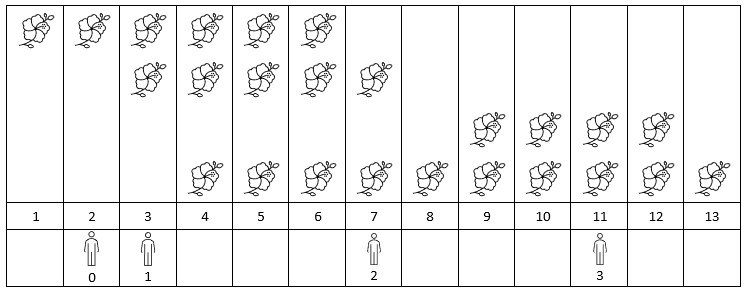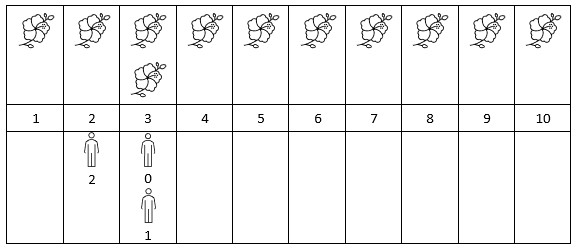##### Welcome to Subscribe On Youtube

Formatted question description: https://leetcode.ca/all/2251.html

# 2251. Number of Flowers in Full Bloom

• Difficulty: Hard.
• Related Topics: Array, Hash Table, Binary Search, Sorting, Prefix Sum, Ordered Set.
• Similar Questions: Meeting Rooms II, Minimum Interval to Include Each Query.

## Problem

You are given a 0-indexed 2D integer array flowers, where flowers[i] = [starti, endi] means the ith flower will be in full bloom from starti to endi (inclusive). You are also given a 0-indexed integer array persons of size n, where persons[i] is the time that the ith person will arrive to see the flowers.

Return an integer array **answer of size n, where answer[i] is the number of flowers that are in full bloom when the ith person arrives.**

Example 1:Input: flowers = [[1,6],[3,7],[9,12],[4,13]], persons = [2,3,7,11]
Output: [1,2,2,2]
Explanation: The figure above shows the times when the flowers are in full bloom and when the people arrive.
For each person, we return the number of flowers in full bloom during their arrival.


Example 2:Input: flowers = [[1,10],[3,3]], persons = [3,3,2]
Output: [2,2,1]
Explanation: The figure above shows the times when the flowers are in full bloom and when the people arrive.
For each person, we return the number of flowers in full bloom during their arrival.


Constraints:

• 1 <= flowers.length <= 5 * 104

• flowers[i].length == 2

• 1 <= starti <= endi <= 109

• 1 <= persons.length <= 5 * 104

• 1 <= persons[i] <= 109

## Solution

• class Solution {
public int[] fullBloomFlowers(int[][] flowers, int[] persons) {
Arrays.sort(flowers, Comparator.comparingInt(a -> a));
int[] ans = new int[persons.length];
PriorityQueue<Pair> pq = new PriorityQueue<>(Comparator.comparingInt(a -> a.j));
int j = 0;
int[][] t = new int[persons.length];
for (int i = 0; i < persons.length; i++) {
t[i] = persons[i];
t[i] = i;
}
Arrays.sort(t, Comparator.comparingInt(a -> a));
for (int[] ints : t) {
while (!pq.isEmpty()) {
if (pq.peek().j < ints) {
pq.poll();
} else {
break;
}
}
while (j < flowers.length) {
if (flowers[j] <= ints && flowers[j] >= ints) {
j++;
continue;
}
if (flowers[j] < ints) {
j++;
continue;
}
break;
}
ans[ints] = pq.size();
}
return ans;
}

private static class Pair {
int i;
int j;

Pair(int i, int j) {
this.i = i;
this.j = j;
}
}
}

• # 2251. Number of Flowers in Full Bloom
# https://leetcode.com/problems/number-of-flowers-in-full-bloom/

class Solution:
def fullBloomFlowers(self, flowers: List[List[int]], persons: List[int]) -> List[int]:
heap = []

for start, end in flowers:
heapq.heappush(heap, (start, 1))
heapq.heappush(heap, (end + 1, -1))

query = [(x, i) for i, x in enumerate(persons)]
query.sort()
n = len(query)
queryIndex = 0
ans =  * n

curr = 0
while queryIndex < n and heap:
while heap and heap <= query[queryIndex]:
x, inc = heapq.heappop(heap)
if inc == -1:
curr -= 1
else:
curr += 1

x, i = query[queryIndex]
ans[i] = curr
queryIndex += 1

return ans


• function fullBloomFlowers(flowers: number[][], persons: number[]): number[] {
// 离散差分
let hashMap = new Map();
for (let [start, end] of flowers) {
end++;
hashMap.set(start, (hashMap.get(start) || 0) + 1);
hashMap.set(end, (hashMap.get(end) || 0) - 1);
}
for (let p of persons) {
if (!hashMap.has(p)) {
hashMap.set(p, 0);
}
}
let keys = Array.from(hashMap.keys()).sort((a, b) => a - b);
let pre = 0;
for (let k of keys) {
pre += hashMap.get(k);
hashMap.set(k, pre);
}
// 离散查询
let ans = persons.map(v => hashMap.get(v));
return ans;
}


• class Solution {
public:
vector<int> fullBloomFlowers(vector<vector<int>>& flowers, vector<int>& people) {
int n = flowers.size();
vector<int> start;
vector<int> end;
for (auto& f : flowers) {
start.push_back(f);
end.push_back(f);
}
sort(start.begin(), start.end());
sort(end.begin(), end.end());
vector<int> ans;
for (auto& p : people) {
auto r = upper_bound(start.begin(), start.end(), p) - start.begin();
auto l = lower_bound(end.begin(), end.end(), p) - end.begin();
ans.push_back(r - l);
}
return ans;
}
};

• func fullBloomFlowers(flowers [][]int, people []int) (ans []int) {
n := len(flowers)
start := make([]int, n)
end := make([]int, n)
for i, f := range flowers {
start[i] = f
end[i] = f
}
sort.Ints(start)
sort.Ints(end)
for _, p := range people {
r := sort.SearchInts(start, p+1)
l := sort.SearchInts(end, p)
ans = append(ans, r-l)
}
return
}

• use std::collections::BTreeMap;

impl Solution {
pub fn full_bloom_flowers(flowers: Vec<Vec<i32>>, people: Vec<i32>) -> Vec<i32> {
let n = people.len();

// First sort the people vector based on the first item
let mut people: Vec<(usize, i32)> = people
.into_iter()
.enumerate()
.map(|x| x)
.collect();

people.sort_by(|lhs, rhs| {
lhs.1.cmp(&rhs.1)
});

// Initialize the difference vector
let mut diff = BTreeMap::new();
let mut ret = vec![0; n];

for f in flowers {
let (left, right) = (f, f);
diff
.entry(left)
.and_modify(|x| *x += 1)
.or_insert(1);

diff
.entry(right + 1)
.and_modify(|x| *x -= 1)
.or_insert(-1);
}

let mut sum = 0;
let mut i = 0;
for (k, v) in diff {
while i < n && people[i].1 < k {
ret[people[i].0] += sum;
i += 1;
}
sum += v;
}

ret
}
}


Explain:

nope.

Complexity:

• Time complexity : O(n).
• Space complexity : O(n).